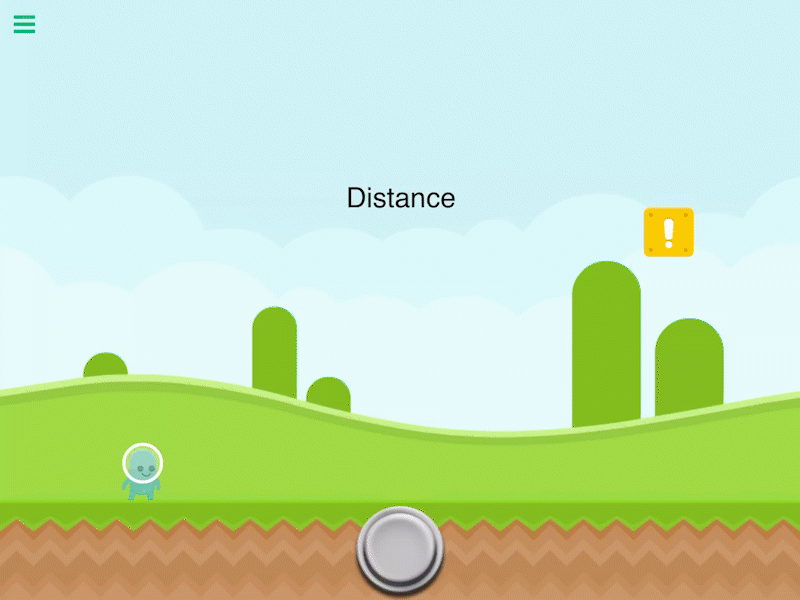# Calculate Distance

Description

Calculate the distance between 2 objects. The distance will be different depending on each zoom. So if you zoom out the distance will be less because it visually looks like there is less distance between them. To compensate for this, you must multiply by the zoom of your screen.

Properties

Triggers Immediately

 Object A By default this is the current object selected. You can change this to any other object in your scene. Object B Another object in your scene to calculate the distance between. Anchor Use the anchor to offset the position. By default the distance is calculated between the center of 2 objects.

Outputs

 Value Outputs a numerical value for the distance between Object A and Object B.

Related

Calculate Direction

Get Bounding Box

Get Time

Is Intersecting